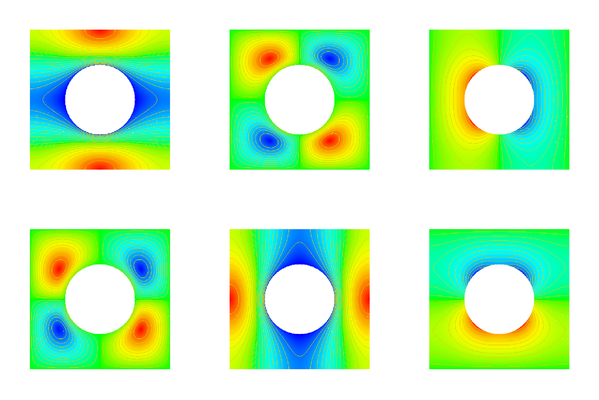Effective permeability

# Calculation of a permeability tensorAssume that we have a porous medium with periodically distributed holes. In this medium we have a flow governed by Stokes equations. On a macroscopic scale the averaged flow is given as div(u)=0 for a velocity field given by u=-K grad p. The tensor K can be calculated by solving a Stokes problem in a representative cell. There are two cell problems to solve (x-direction and y-direction), each having three components (speed in x- and y-direction and pressure). These are shown in the above picture.

The permeability tensor is computed averages the velocities of those cell solutions. In our case, the cell is symmetric, thus the permeability tensor is isotropic and determined by a scalar K.

We have solved this problem using mixed (Q5)2/Q4 finite elements. Linear solver was a W(1,1)-multigrid cycle with a vertex-centered subspace correction scheme as smoother. Convergence rates were generally smaller than 0.05.

The history of this calculation was as follows:

Cells Unknowns Matrix entries K
4 578 33823 1.9943087655d-02
16 2218 142179 1.9901508352d-02
64 8666 567991 1.9901435210d-02
256 34234 2267511 1.9901435350d-02

Nicolas Neuss, 2017-01-31